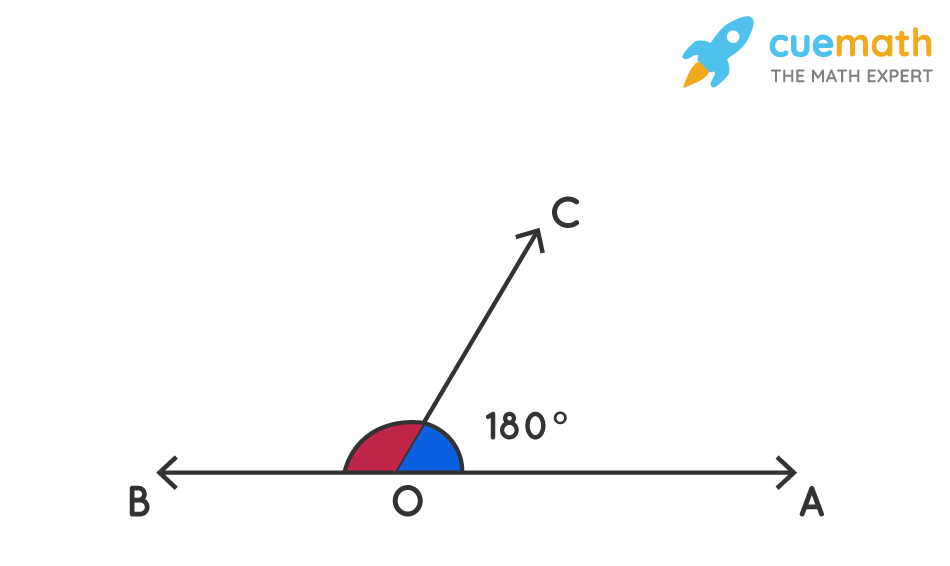# If the Exterior Sides of Two Adjacent Angles Are Opposite Rays, Then the Angles Are _____ Angles.

## Question: If the exterior sides of two adjacent angles are opposite rays, then the angles are _____ angles.

If the sum of two adjacent angles is 1800,  then the non-common arms of the angles form a line. If a ray stands on a line, the sum of the pair of adjacent angles is 1800.

## Answer: If the exterior sides of two adjacent angles are opposite rays, then the angles are supplementary angles.

Let us understand the solution with a suitable diagram.

## Explanation:

If the exterior sides of two adjacent angles are opposite rays, then the angles are supplementary angles.

• Two angles are said to be supplementary if the sum of both the angles is 180°.
• If the two supplementary angles are adjacent to each other then they are called a linear pair.
• That means they have a common vertex and a common ray. The non-common rays are opposite rays.Here, OA and OB are opposite rays. O is the common vertex and OC is the common ray.

⇒ ∠BOC + ∠AOC = 180°